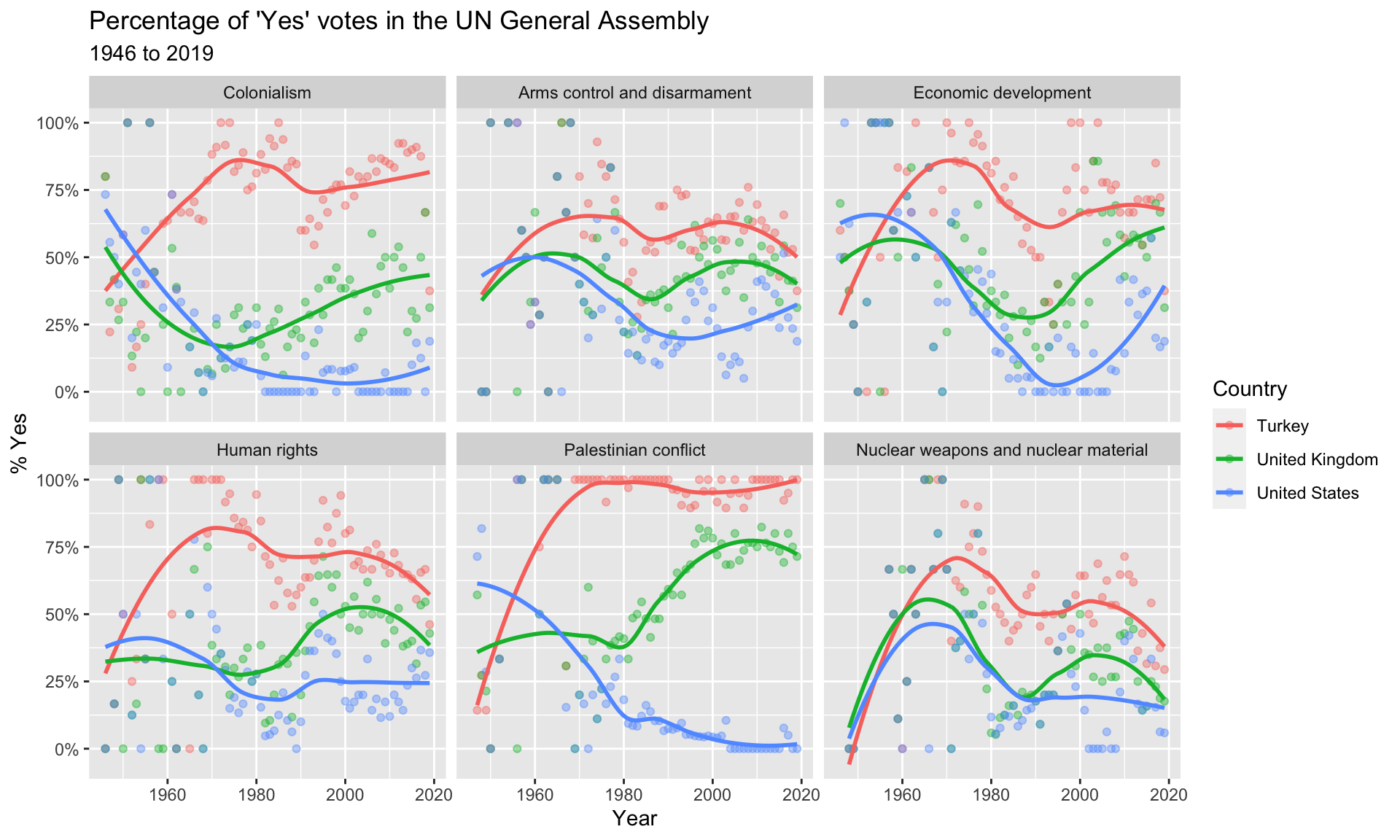Author

Mine Çetinkaya-Rundel

## Introduction

How do various countries vote in the United Nations General Assembly, how have their voting patterns evolved throughout time, and how similarly or differently do they view certain issues? Answering these questions (at a high level) is the focus of this analysis.

### Packages

We will use the tidyverse, lubridate, and scales packages for data wrangling and visualization, and the DT package for interactive display of tabular output, and the unvotes package for the data.

``````library(tidyverse)
library(lubridate)
library(scales)
library(DT)

### Data

The data we’re using originally come from the unvotes package. In the chunk below we modify the data by joining the various data frames provided in the package to help you get started with the analysis.

``````unvotes <- un_votes %>%
inner_join(un_roll_calls, by = "rcid") %>%
inner_join(un_roll_call_issues, by = "rcid")``````

## UN voting patterns

Let’s create a data visualisation that displays how the voting record of the UK & NI changed over time on a variety of issues, and compares it to two other countries: US and Turkey.

We can easily change which countries are being plotted by changing which countries the code above `filter`s for. Note that the country name should be spelled and capitalized exactly the same way as it appears in the data. See the Appendix for a list of the countries in the data.

``````unvotes %>%
filter(country %in% c("United Kingdom", "United States", "Turkey")) %>%
mutate(year = year(date)) %>%
group_by(country, year, issue) %>%
summarize(percent_yes = mean(vote == "yes")) %>%
ggplot(mapping = aes(x = year, y = percent_yes, color = country)) +
geom_point(alpha = 0.4) +
geom_smooth(method = "loess", se = FALSE) +
facet_wrap(~issue) +
scale_y_continuous(labels = percent) +
labs(
title = "Percentage of 'Yes' votes in the UN General Assembly",
subtitle = "1946 to 2019",
y = "% Yes",
x = "Year",
color = "Country"
)``````## Appendix

Below is a list of countries in the dataset: